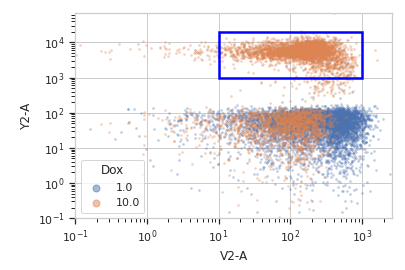# cytoflow.operations.range2d¶

class cytoflow.operations.range2d.Range2DOp[source]

Apply a 2D range gate to a cytometry experiment.

name

The operation name. Used to name the new metadata field in the experiment that’s created by apply()

Type: Str
xchannel

The name of the first channel to apply the range gate.

Type: Str
xlow

The lowest value in xchannel to include in this gate.

Type: Float
xhigh

The highest value in xchannel to include in this gate.

Type: Float
ychannel

The name of the secon channel to apply the range gate.

Type: Str
ylow

The lowest value in ychannel to include in this gate.

Type: Float
yhigh

The highest value in ychannel to include in this gate.

Type: Float

Examples

Make a little data set.

>>> import cytoflow as flow
>>> import_op = flow.ImportOp()
>>> import_op.tubes = [flow.Tube(file = "Plate01/RFP_Well_A3.fcs",
...                              conditions = {'Dox' : 10.0}),
...                    flow.Tube(file = "Plate01/CFP_Well_A4.fcs",
...                              conditions = {'Dox' : 1.0})]
>>> import_op.conditions = {'Dox' : 'float'}
>>> ex = import_op.apply()


Create and parameterize the operation.

>>> r = flow.Range2DOp(name = "Range2D",
...                    xchannel = "V2-A",
...                    xlow = 10,
...                    xhigh = 1000,
...                    ychannel = "Y2-A",
...                    ylow = 1000,
...                    yhigh = 20000)


Show the default view.

>>> rv = r.default_view(huefacet = "Dox",
...                     xscale = 'log',
...                     yscale = 'log')

>>> rv.plot(ex)Note

If you want to use the interactive default view in a Jupyter notebook, make sure you say %matplotlib notebook in the first cell (instead of %matplotlib inline or similar). Then call default_view() with interactive = True:

rv = r.default_view(huefacet = "Dox",
xscale = 'log',
yscale = 'log',
interactive = True)
rv.plot(ex)


Apply the gate, and show the result

>>> ex2 = r.apply(ex)
>>> ex2.data.groupby('Range2D').size()
Range2D
False    16405
True      3595
dtype: int64

apply(experiment)[source]

Applies the threshold to an experiment.

Parameters: experiment (Experiment) – the old_experiment to which this op is applied a new Experiment, the same as the old experiment but with a new column with a data type of bool and the same as the operation name. The bool is True if the event’s measurement in xchannel is greater than xlow and less than high, and the event’s measurement in ychannel is greater than ylow and less than yhigh; it is False otherwise. Experiment
default_view(**kwargs)[source]
class cytoflow.operations.range2d.RangeSelection2D[source]

Plots, and lets the user interact with, a 2D selection.

interactive

is this view interactive? Ie, can the user set min and max with a mouse drag?

Type: Bool
xchannel, ychannel

The channels to use for this view’s X and Y axes. If you created the view using default_view(), this is already set.

Type: String
xscale, yscale

The way to scale the x axes. If you created the view using default_view(), this may be already set.

Type: {‘linear’, ‘log’, ‘logicle’}
op

The IOperation that this view is associated with. If you created the view using default_view(), this is already set.

Type: Instance(IOperation)
xlim, ylim

Set the min and max limits of the plots’ x and y axes.

Type: (float, float)
xfacet, yfacet

Set to one of the conditions in the Experiment, and a new row or column of subplots will be added for every unique value of that condition.

Type: String
huefacet

Set to one of the conditions in the in the Experiment, and a new color will be added to the plot for every unique value of that condition.

Type: String
huescale

How should the color scale for huefacet be scaled?

Type: {‘linear’, ‘log’, ‘logicle’}

Notes

We inherit xfacet and yfacet from cytoflow.views.ScatterplotView, but they must both be unset!

Examples

In a Jupyter notebook with %matplotlib notebook

>>> r = flow.Range2DOp(name = "Range2D",
...                    xchannel = "V2-A",
...                    ychannel = "Y2-A"))
>>> rv = r.default_view()
>>> rv.interactive = True
>>> rv.plot(ex2)

plot(experiment, **kwargs)[source]

Plot the underlying scatterplot and then plot the selection on top of it.

Parameters: experiment (Experiment) – The Experiment to plot using this view. title (str) – Set the plot title xlabel, ylabel (str) – Set the X and Y axis labels huelabel (str) – Set the label for the hue facet (in the legend) legend (bool) – Plot a legend for the color or hue facet? Defaults to True. sharex, sharey (bool) – If there are multiple subplots, should they share axes? Defaults to True. row_order, col_order, hue_order (list) – Override the row/column/hue facet value order with the given list. If a value is not given in the ordering, it is not plotted. Defaults to a “natural ordering” of all the values. height (float) – The height of each row in inches. Default = 3.0 aspect (float) – The aspect ratio of each subplot. Default = 1.5 col_wrap (int) – If xfacet is set and yfacet is not set, you can “wrap” the subplots around so that they form a multi-row grid by setting col_wrap to the number of columns you want. sns_style ({“darkgrid”, “whitegrid”, “dark”, “white”, “ticks”}) – Which seaborn style to apply to the plot? Default is whitegrid. sns_context ({“paper”, “notebook”, “talk”, “poster”}) – Which seaborn context to use? Controls the scaling of plot elements such as tick labels and the legend. Default is talk. despine (Bool) – Remove the top and right axes from the plot? Default is True. min_quantile (float (>0.0 and <1.0, default = 0.001)) – Clip data that is less than this quantile. max_quantile (float (>0.0 and <1.0, default = 1.00)) – Clip data that is greater than this quantile. xlim, ylim ((float, float)) – Set the range of the plot’s axis. alpha (float (default = 0.25)) – The alpha blending value, between 0 (transparent) and 1 (opaque). s (int (default = 2)) – The size in points^2. marker (a matplotlib marker style, usually a string) – Specfies the glyph to draw for each point on the scatterplot. See matplotlib.markers for examples. Default: ‘o’